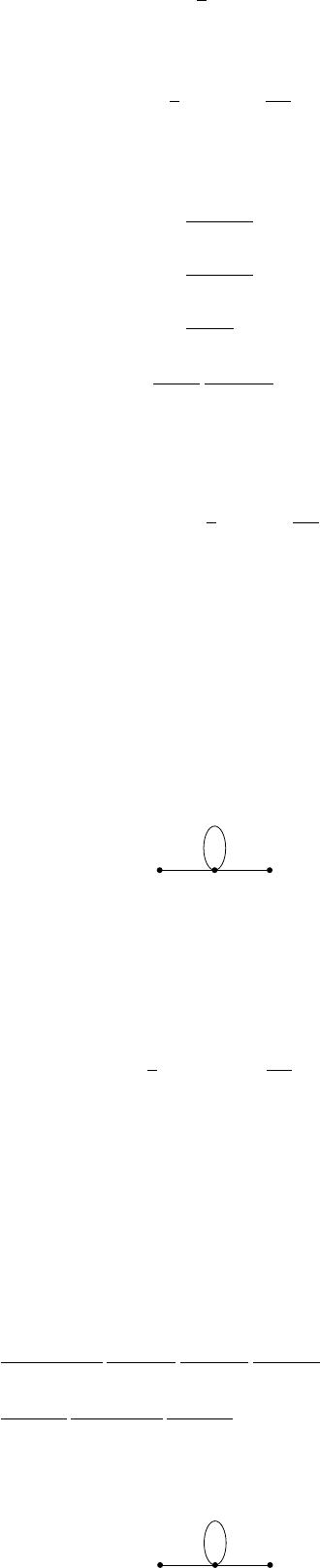2QFT in one dimension (i.e. QM)2.4 Quantum gravity in one dimension
In quantum gravity, we also include a (path) integral over all metrics on our
spacetime, up to diffeomorphism (isometric) invariance. We also sum over all
possible topologies of
M
. In
d
= 1 (and
d
= 2 for string theory), we can just do
this.
In
d
= 1, a metric
g
only has one component
g
tt
(
t
) =
e
(
t
). There is no
curvature, and the only diffeomorphism invariant of this metric is the total
length
T =
I
e(t) dt.
So the instruction to integrate over all metrics modulo diffeomorphism is just
the instruction to integrate over all possible lengths of the worldline
T
(0
,
),
which is easy. Let’s look at that.
The path integral is given by
Z
T
dT
Z
C
[0,T ]
[y,x]
Dx e
S[x]
.
where as usual
S[x] =
1
2
Z
T
0
˙x
2
dt.
Just for fun, we will include a “cosmological constant” term into our action, so
S[x] =
1
2
Z
T
0
˙x
2
+
m
2
2
dt.
The reason for this will be revealed soon.
We can think of the path integral as the heat kernel, so we can write it as
Z
0
dT hy|e
HT
|xi =
Z
0
dT
d
n
p d
n
q
(2π)
n
hy|qihq|e
HT
|pihp|xi
=
Z
0
dT
d
n
p d
n
q
(2π)
n
e
ip·xiq·y
e
T (p
2
+m
2
)/2
δ
n
(p q)
=
Z
0
dT
d
n
p
(2π)
n
e
ip·(xy)
e
T (p
2
+m
2
)/2
= 2
Z
d
n
p
(2π)
n
e
ip·(xy)
p
2
+ m
2
= 2D(x, y),
where
D
(
x, y
) is the propagator for a scalar field on the target space
R
n
with
action
S[Φ] =
Z
d
n
x
1
2
(Φ)
2
+
m
2
2
Φ
2
.
So a 1-dimensional quantum gravity theory with values in
R
n
is equivalent to
(or at least has deep connections to) a scalar field theory on R
n
.
How about interactions? So far, we have been taking rather unexciting
1-dimensional manifolds as our universe, and there are only two possible choices.
If we allow singularities in our manifolds, then we would allow graphs instead of
just a line and a circle. Quantum gravity then says we should not only integrate
over all possible lengths, but also all possible graphs.
For example, to compute correlation functions such as
h
Φ(
x
1
)
···
Φ(
x
n
)
i
in
Φ
4
theory on
R
n
, say, we consider all 4-valent with
n
external legs with one
endpoint at each of the x
i
, and then we proceed just as in quantum gravity.
For example, we get a contribution to hΦ(x)Φ(y)i from the graph
x x
The contribution to the quantum gravity expression is
Z
zR
n
d
n
z
Z
[0,)
3
dT
1
dT
2
dT
3
Z
C
T
1
[z,x]
Dx e
S
T
1
[x]
Z
C
T
2
[z,z]
Dx e
S
T
2
[x]
Z
C
T
3
[y,z]
Dx e
S
T
3
[x]
,
where
S
T
[x] =
1
2
Z
T
0
x
2
dt +
m
2
2
Z
T
0
dt.
We should think of the second term as the “cosmological constant”, while the
1D integrals over
T
i
’s are the “1d quantum gravity” part of the path integral
(also known as the Schwinger parameters for the graph).
We can write this as
Z
d
n
z dT
1
dT
2
dT
3
hz|e
HT
1
|xihz|e
HT
2
|zihy|e
HT
3
|zi.
Inserting a complete set of eigenstates between the position states and the time
evolution operators, we get
=
Z
d
n
p d
n
` d
n
q
(2π)
3n
e
ip·(xz)
p
2
+ m
2
e
iq·(yz)
q
2
+ m
2
e
i`·(zz)
`
2
+ m
2
=
Z
d
n
p d
n
`
(2π)
2n
e
ip·(xy)
(p
2
+ m
2
)
2
1
`
2
+ m
2
.
This is exactly what we would have expected if we viewed the above diagram as
a Feynman diagram:
x
y
p p
`
This is the worldline perspective to QFT, and it was indeed Feynman’s original
approach to doing QFT.